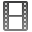TOP > Movie Library > Reference electrode introduction

Introduction of the reference electrode

BAS has a wide line of the reference electrode, as reference electrode for measurement in aqueous solution, non-aqueous solution, alkaline solution, and hydrogen electrode.
Here we will summarized the features of the products, how to use, and some other advice.Download the reference electrode introduction movie

Topics of the movie:

1. What is three electrode method?
2. Types of reference electrode.
3. Introduction for optional items.
4. Method of reference electrode storage.
5. Example of reference electrode damage
6. How to check the reference electrode?
7. Replacement timing of the reference electrode